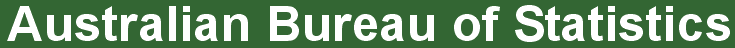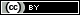ABS Home
 Understanding statistics

Statistical Language helps you to understand a range of statistical concepts and terms with simple explanations.

Find concept definitions:Statistical Language Glossary
Explore a concept:What are Data? - Data unit - Data item (variable) - Observation - DatasetQuantitative and Qualitative Data - Quantitative data - Qualitative dataWhat are Variables? - Variable (data item) - Numeric - Continuous - Discrete - Categorical - Ordinal - NominalWhat is a Population? - PopulationCensus and Sample - Census - Sample - Random (probability) sample - Non-random (non-probability) sampleData Sources - Direct/Primary data - Survey - Indirect/Secondary data - Administrative dataDescribing Frequencies - Absolute frequency - Relative frequency - Ratio - Rate - ProportionFrequency Distribution - Frequency distribution - Histogram - Bar chartMeasures of Shape - Measures of shape - Normal distribution - SkewnessMeasures of Central Tendency - Mode - Median - Mean - OutlierMeasures of Spread - Range - Quartiles - Interquartile range - Variance - Standard deviationTypes of Error - Sampling error - Non-sampling errorMeasures of Error - Standard error (SE) - Relative error (RE) - Confidence intervalWhat are Statistics? - Descriptive (summary) statistics - Inferential statisticsWhat are Standards? - Statistical standard - ClassificationWhat is Metadata? - MetadataData Visualisation - Static - Dynamic - InteractiveTime Series Data - Original time series - Seasonally adjusted time series - Trend seriesEstimate and Projection - Estimate - ProjectionCorrelation and Causation - Correlation - Causation (Causality)Confidentiality - Confidentiality

 Privacy | Disclaimer | Feedback || © Copyright| Sitemap| Online Security